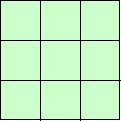#### You may also like### Prompt Cards

These two group activities use mathematical reasoning - one is numerical, one geometric.### Consecutive Numbers

An investigation involving adding and subtracting sets of consecutive numbers. Lots to find out, lots to explore.### Exploring Wild & Wonderful Number Patterns

EWWNP means Exploring Wild and Wonderful Number Patterns Created by Yourself! Investigate what happens if we create number patterns using some simple rules.

# 1, 2, 3 Magic Square

## 1, 2, 3 Magic Square

Arrange three $1$s, three $2$s and three $3$s in this square so that every row, column and diagonal adds to the same total.You might like to use this interactivity to do this problem.

You may like to try this with other sets of three consecutive numbers (numbers which come one after the other).

This problem has been adapted from the book "Numbers in Your Head" by John Spooner, published by BEAM Education. This book is out of print but can still be found on Amazon.

### Why do this problem?

This problem requires only simple adding, but also persistence and logical thinking.

### Key questions

What do one, two and three add to?
Which of the numbers, when multiplied by three, will come to the total you want in each of the rows?
What do three twos add to?
How many completely different solutions can you find?

### Possible extension

Learners could try this activity with other sets of three consecutive numbers.

### Possible support

Suggest using the interactivity or counters marked appropriately on a $3 \times 3$ square.